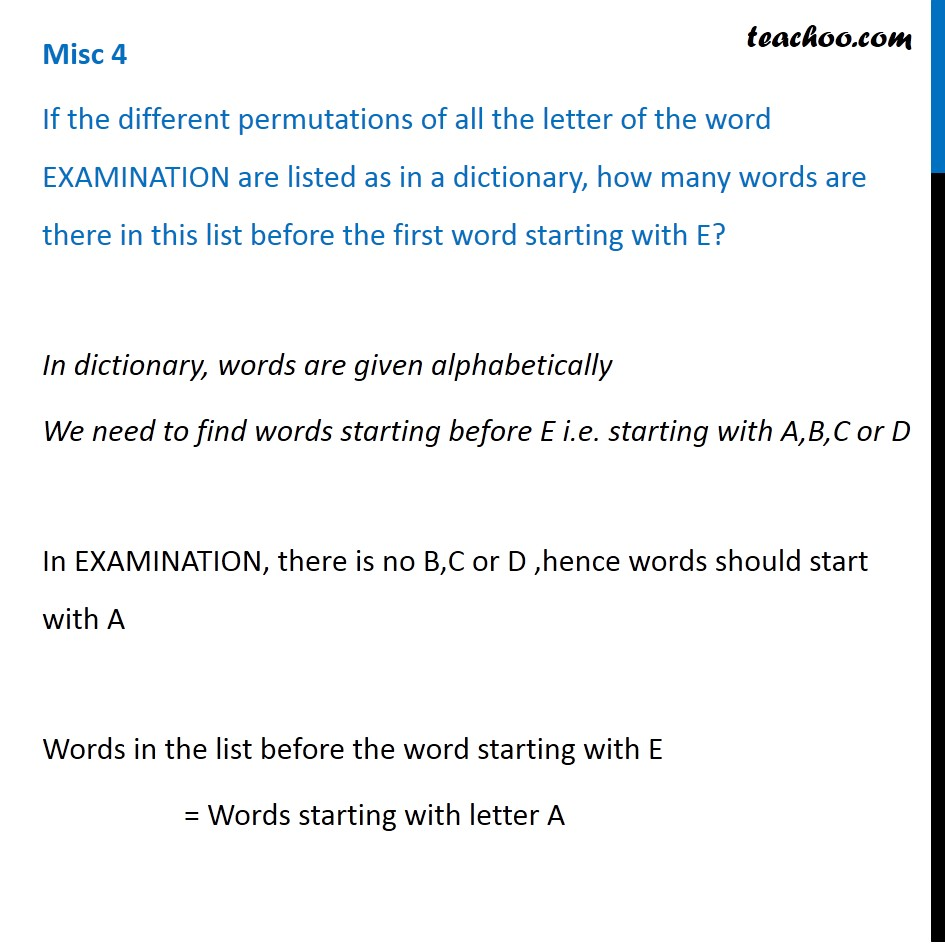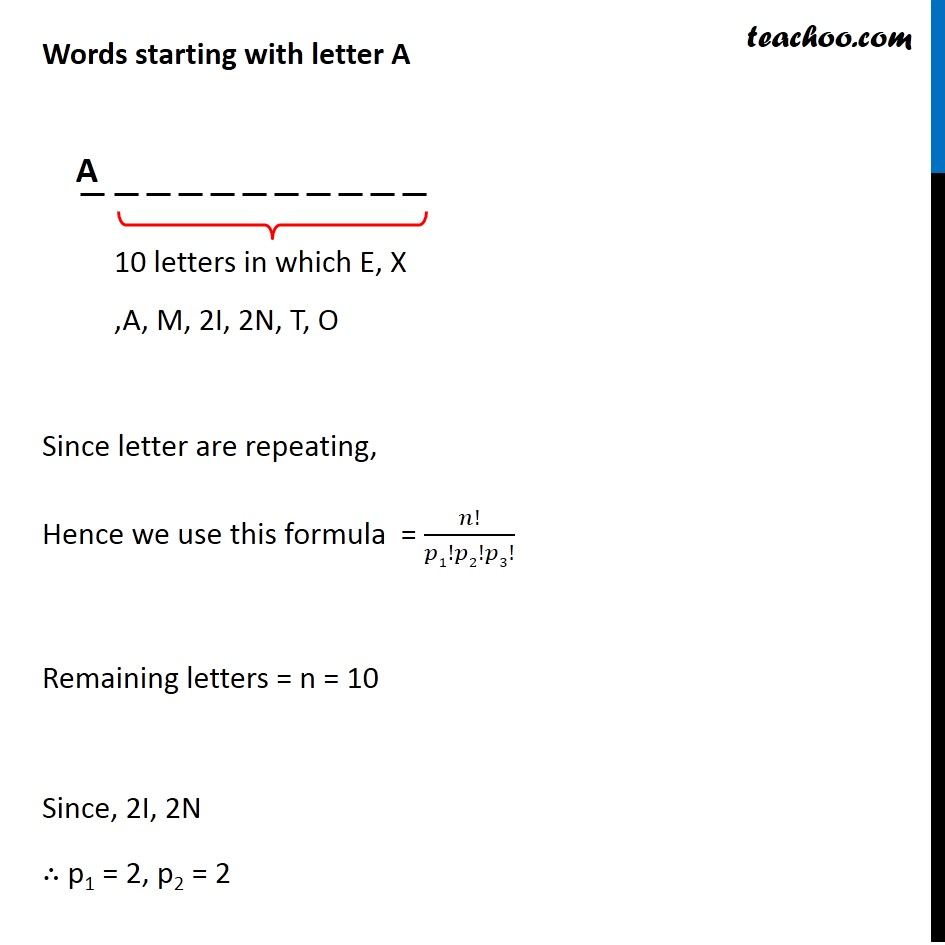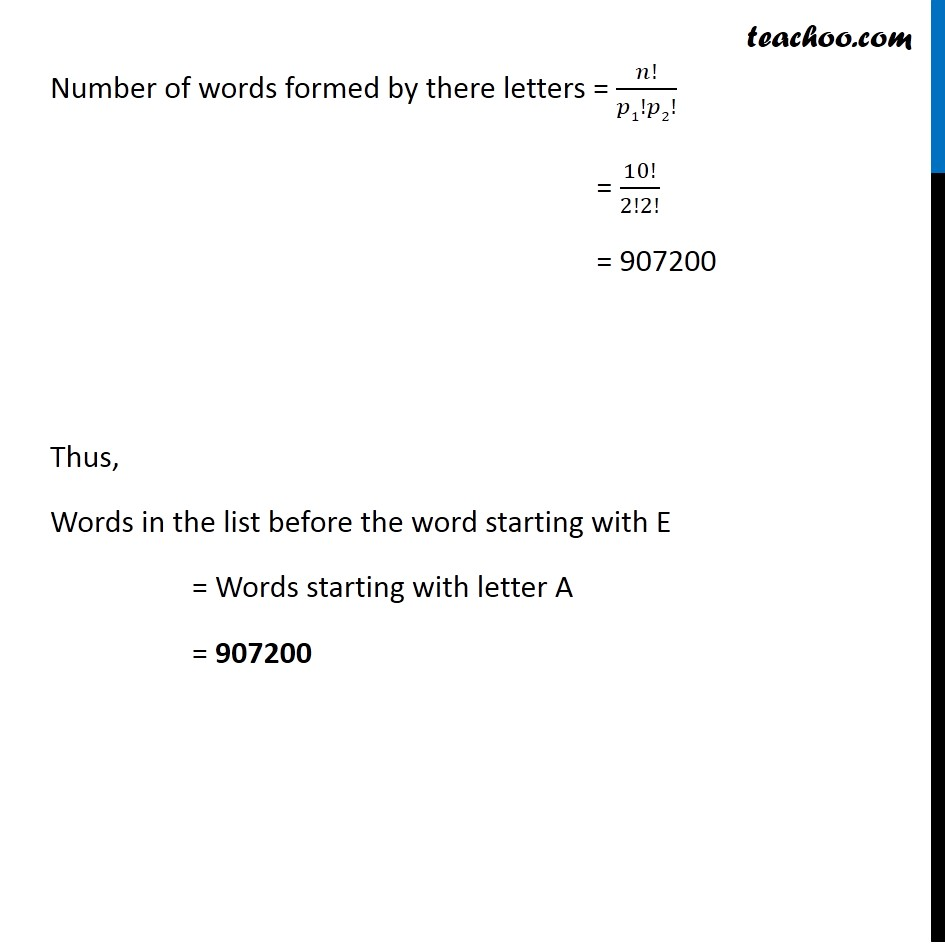Miscellaneous

Chapter 6 Class 11 Permutations and Combinations
Serial order wiseLearn in your speed, with individual attention - Teachoo Maths 1-on-1 Class

### Transcript

Misc 4 If the different permutations of all the letter of the word EXAMINATION are listed as in a dictionary, how many words are there in this list before the first word starting with E? In dictionary, words are given alphabetically We need to find words starting before E i.e. starting with A,B,C or D In EXAMINATION, there is no B,C or D ,hence words should start with A Words in the list before the word starting with E = Words starting with letter A Words starting with letter A Since letter are repeating, Hence we use this formula = 𝑛!/𝑝1!𝑝2!𝑝3! Remaining letters = n = 10 Since, 2I, 2N ∴ p1 = 2, p2 = 2 Words starting with letter A Since letter are repeating, Hence we use this formula = 𝑛!/𝑝1!𝑝2!𝑝3! Remaining letters = n = 10 Since, 2I, 2N ∴ p1 = 2, p2 = 2 Number of words formed by there letters = 𝑛!/𝑝1!𝑝2! = 10!/2!2! = 907200 Thus, Words in the list before the word starting with E = Words starting with letter A = 907200